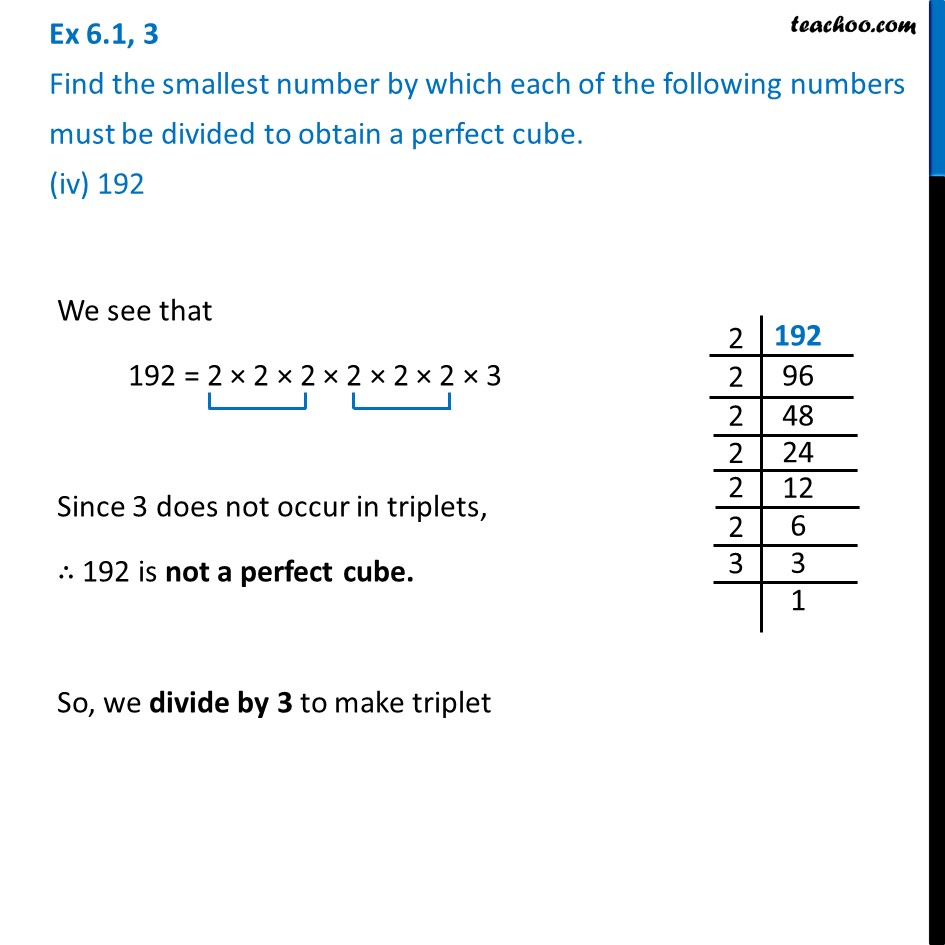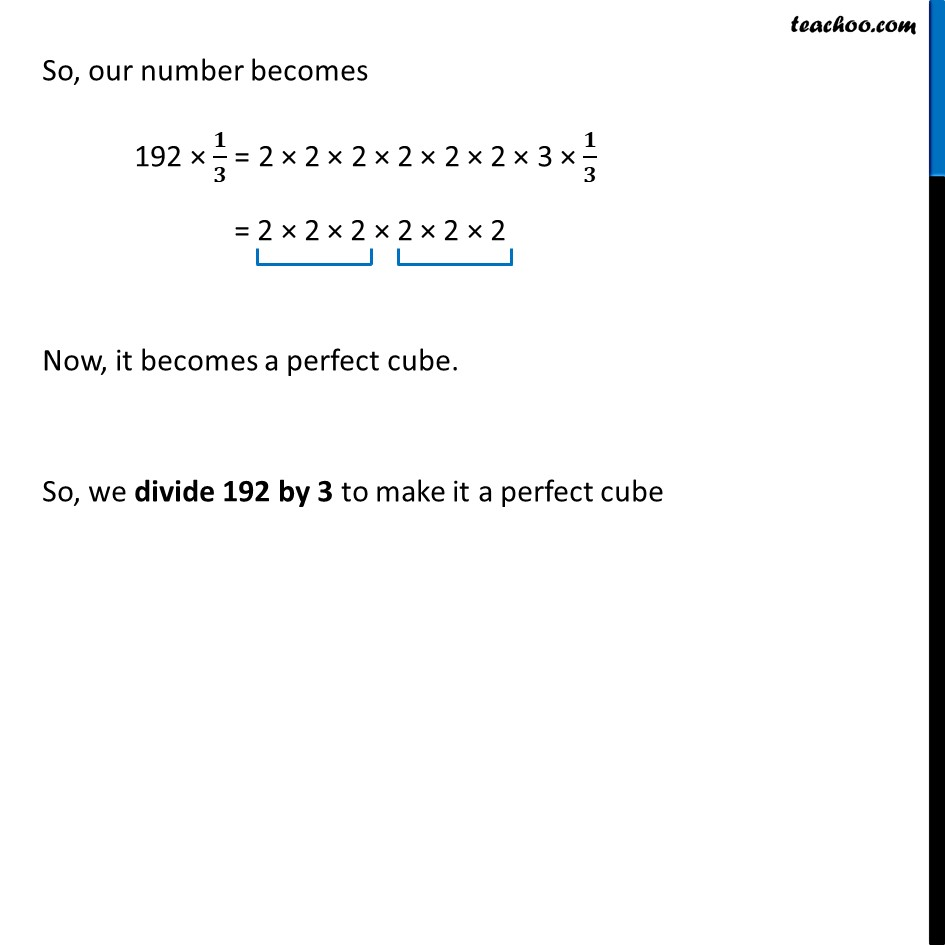Ex 6.1

Chapter 6 Class 8 Cubes and Cube Roots
Serial order wiseLearn in your speed, with individual attention - Teachoo Maths 1-on-1 Class

### Transcript

Ex 6.1, 3 Find the smallest number by which each of the following numbers must be divided to obtain a perfect cube. (iv) 192We see that 192 = 2 × 2 × 2 × 2 × 2 × 2 × 3 Since 3 does not occur in triplets, ∴ 192 is not a perfect cube. So, we divide by 3 to make triplet So, our number becomes 192 × 𝟏/𝟑 = 2 × 2 × 2 × 2 × 2 × 2 × 3 × 𝟏/𝟑 = 2 × 2 × 2 × 2 × 2 × 2 Now, it becomes a perfect cube. So, we divide 192 by 3 to make it a perfect cube